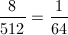Example Questions

Example Question #51 : Probability

A game is played where players take turns rolling two six-sided dice, each with sides numbered 1-6.  The numbers on the two dice are added together for each player's score.  If the first player rolls a sum of 10, what is the probability that the second player rolls a HIGHER total?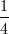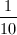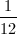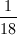Explanation:

A roll of two 6-sided dice yields 36 total possible outcomes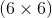. There are three possible ways to roll a sum higher than 10:

5, 6

6, 5

6, 6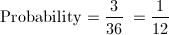Example Question #51 : Probability

10 cards, each with a distinct number from 1-10, are placed face-down on a table.  If Matt chooses a card at random, what is the probability his card will display a prime number?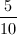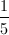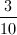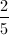Explanation:

Total outcomes: 10

Desired outcomes (prime numbers) = 4   (2, 3, 5, 7)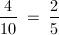Example Question #53 : Probability

A coin with two sides, heads and tails, is flipped repeatedly and the result recorded. Assuming an equal chance of it landing either way, what is the probability of each of the first four flips being heads?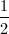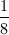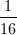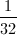Explanation: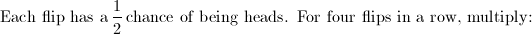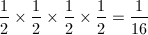Example Question #54 : Probability

A coin with two sides, heads and tails, is flipped 10 times and the results recorded.  What is the probability that the 10th flip results in tails?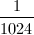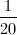Explanation: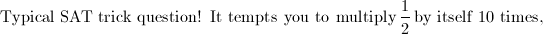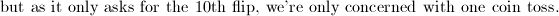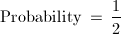Example Question #55 : Probability

A dresser drawer contains only 4 blue socks, 6 white socks, and 2 red socks.  If two socks are pulled from the drawer at random, what is the probability that they are both white?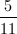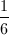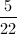Explanation:

For this question to be satsified, both socks drawn must be white.

First draw:  6 white socks out of 12 total: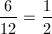Second draw: 5 white socks out of 11 total:Multiply together to find the answer: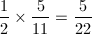Example Question #56 : Probability

In a hotel with 120 rooms, 30 of them have red carpet.  A hotel manager has lost his keys somewhere in the hotel.  If there is an equal chance of the keys being in any room, what is the probability that they are in a room with red carpet?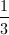Explanation:

Probability = desired outcomes divided by total possible outcomes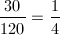Example Question #57 : Probability

A bag contains the following numbers of apples:  12 red delicious, 10 granny smith, and 7 fuji apples.  If an apple is selected at random, what is the probability it is either a granny smith or a fuji apple?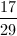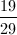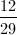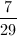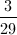Explanation:

Probability = desired outcomes divided by total possible outcomes. Desired outcome can be either fuji or granny smith.

Desired outcomes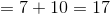Total outcomes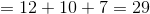Example Question #58 : Probability

From a class consisting of 15 girls and 12 boys, two students are chosen at random to be class representatives.  What is the probability that neither of the two students chosen is a boy?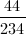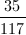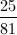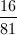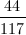Explanation:

To satisfy the question, BOTH students chosen must be girls.

First selection: 15 girls of 27 total students: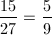Second selection: 14 girls out of 26 total students: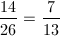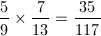Example Question #59 : Probability

If 2 six-sided dice, each with sides numbered 1-6, are rolled, what is the probability that the sum of the numbers on the face-up sides is equal to 7?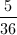Explanation:

Probability = desired outcomes divided by total possible outcomes.

Desired outcomes = 6   (1,6; 2,5; 3,4; 4,3; 5,2; 6;1)

Total outcomes - 36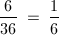Example Question #60 : Probability

If an 8-sided die is rolled 3 times, what is the probability that the same number will be rolled each time?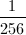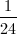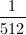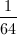Explanation: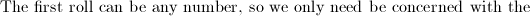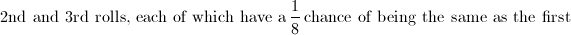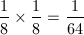Another way to look a it is there are 512 total possible outcomes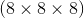and 8 desired outcomes.